长沙小升初数学择校考试速算口诀长沙奥数网6月13日：在长沙小升初数学择校考试中，由于各方面的原因，导致有时候考生需要在考试时间不充足的情况下，完成本场考试，在这个情况下，如果计算方面能够提高速度，对孩子的考试是有百利而无一害的。

1、十几乘十几：
口诀：头乘头，尾加尾，尾乘尾。
例：12×14=？
解:1×1=1
2＋4＝6
2×4＝8
12×14=168
注：个位相乘，不够两位数要用0占位。

2、头相同，尾互补(尾相加等于10)：
口诀：一个头加１后，头乘头，尾乘尾。
例：23×27=？
解：２＋１＝３
2×3＝6
3×7＝21
23×27=621
注：个位相乘，不够两位数要用0占位。

3、第一个乘数互补，另一个乘数数字相同：
口诀：一个头加1后，头乘头，尾乘尾。
例：37×44=？
解：3+1=4
4×4=16
7×4=28
37×44=1628
注：个位相乘，不够两位数要用0占位。

4、几十一乘几十一：
口诀：头乘头，头加头，尾乘尾。
例：21×41=？
解：2×4=8
2+4=6
1×1=1
21×41=861

5、11乘任意数：
口诀：首尾不动下落，中间之和下拉。
例：11×23125=？
解：2+3=5
3+1=4
1+2=3
2+5=7
2和5分别在首尾
11×23125=254375
注：和满十要进一。

6、十几乘任意数：
口诀：第二乘数首位不动向下落，第一因数的个位乘以第二因数后面每一个数字，加下一位数，
再向下落。
例：13×326=？
解：13个位是3
3×3+2=11
3×2+6=12
3×6=18
13×326=4238
注：和满十要进一。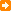您可能还对以下内容感兴趣攻克长沙小升初择校中的数学四大知识点长沙小升初数学思维训练八大解题类型长沙“小升初”奥数学习零失分宝典您还可以通过手机、平板电脑等移动设备访问长沙奥数网，升学路上有我们相伴。>>[点击查看]

•欢迎扫描二维码
关注奥数网微信
ID：aoshu_2003

•欢迎扫描二维码
关注中考网微信
ID：zhongkao_com

教育导航

1.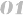北京站 上海站 广州站 深圳站
2.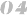天津站 武汉站 成都站
3.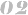南京站 杭州站 济南站 苏州站
4.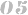郑州站 沈阳站 太原站 重庆站
5.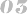长沙站 合肥站 宁波站 青岛站
6.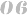石家庄站